# Nanofluid effect on forced convective heat transfer inside a heated horizontal tube

Nanofluid effect on forced convective heat transfer inside a heated horizontal tube

ICB UMR 6303, CNRS, Univ. Bourgogne Franche-Comté, UTBM, Département COMM, F-90010 Belfort, France

Laboratory of Metrology and ENIM, 5000 Monastir, Tunisia

Corresponding Author Email:
mariem.elahmer@utbm.fr
Page:
874-882
|
DOI:
https://doi.org/10.18280/ijht.350424
|
Accepted:
|
Published:
31 December 2019
| Citation

OPEN ACCESS

Abstract:

In this paper, an unsteady forced convection of two-dimensional flow of Ethylene Glycol/CNT and Ethylene Glycol/CNT–Ag in horizontal cylinder is studied. The wall is submitted to a uniform or periodic heat flux. The transient distribution of the temperature is obtained by solving, at each axial position and time, a tridiagonal system by TDMA algorithm. The convective heat transfer coefficient and bulk temperature of nanofluid are investigated versus space-time and versus different nanoparticles volume fraction. The thermal phase shift is brought out for Ethylene Glycol base fluid and EG-CNT nanofluid. The obtained results show an improving of heat transfer rate when the volume fraction increases from 0 % to 10 % of CNT. Ethylene Glycol/CNT-AG seems to be more advantageous for the enhancement of convective heat transfer than EG/CNT. The results found can be exploited for various applications especially for the enhancement performances of heat transfer rate for the absorber tube of solar collector.

Keywords:

forced convection, laminar flow, unsteady, hybrid nanofluid, conjugated heat transfer​

1. Introduction

Fluids such as water, Ethylene Glycol and oils have low thermophysical properties . So, nanofluids have been exhibited novel and improved heat transfer due to their nanoscale size. For this reason, in the past years many experiments have been conducted numerically and analytically to validate the importance of nanofluids as working fluid. Almost all published papers in nanofluids field until nowadays studied the effect of concentration, shape, size, types of nanoparticles and its influence on heat transfer [2-3-4-5]. Maxwell [6-7] was the first who showed the possibility of enhancement of thermal conductivity of a solid-liquid mixture by increasing the volume fraction ratio of solid particles. In fact, Choi and Estman  presented for the first time a suspension of nanoparticles titled nanofluids and its benefit on different thermal systems to improve the heat transfer rate.

As a result, the first study on convective heat transfer of nanofluids in a circular tube was presented by Pak and Cho

The results show that the Nusselt number increases with the increasing of volume fraction of nanoparticles. They proposed also a new correlation for the turbulent flow. Heris et al [10-11] investigated experimentally the convective heat transfer of CuO-water and Al2O3-water nanofluids with different concentrations in annular tube and laminar flow

under a constant wall temperature boundary condition. Also, Heris et al  studied numerically laminar convective heat transfer of nanofluid with constant wall temperature boundary condition through a circular tube. The results show that the heat transfer enhancement appears to be more pronounced with increase of nanoparticle volume fraction and the Nusselt number decreases with the increasing of nanoparticle size. The comparison of the numerical and experimental data shows good agreement.

Many researchers have focused on how to improve the efficiency of the solar collector experimentally. Onticar  has investigated experimentally the effect of different nanofluids as an absorbing medium on the efficiency of the micro solar thermal collector. The increased efficiency was 5%. Yousefi et al  has studied experimentally the effect of Al2O3-water nanofluid on the efficiency of a flat-plate solar collector. The results show that an efficiency improvement up to 28.3% for 0.2 wt% volume fraction of nanoparticles in nanofluid. Lenert and Wang  evaluated the influence of different variations in nanofluid volumetric receivers both experimentally and theoretically. Their results show that the efficiency of nanofluid volumetric receivers is increased with increasing solar concentration and nanofluid high.

Maiga et al  investigated numerically the thermal performance of nanofluid inside a uniformly heated tube. The

flow is assumed as turbulent. The results show the heat transfer with the presence of nanofluid increase. A new correlation of Nusselt number is proposed to calculate the fully developed heat transfer coefficient for the nanofluid.

Maiga et al  studied numerically forced laminar convection flow using water/Al2O3 and Ethylene Glycol/Al2O3nanofluids. Results show that the Ethylene Glycol/Al2O3 nanofluid has a higher heat transfer enhancement than water/Al2O3. So, the Ethylene Glycol is more advantageous than water. M.Izadi et al  studied numerically convective heat transfer of water/Al2O3 nanofluid in horizontal annulus where the wall is submitted to a uniform heat flux. Their results demonstrate that convection heat transfer coefficient and the nanofluid bulk temperature significantly increase with increasing of the nanoparticle volume fractions. Using the two-phase mixture model, Labib et al  developed a forced convection heat transfer study in which they showed that Ethylene Glycol/CNT-Al2O3 which gives better heat transfer enhancement than water/CNT-Al2O3. Moghadassi et Al  studied numerically the effect of Water/ Al2O3 nanofluid and Water/ Al2O3-Cu hybrid nanofluid on laminar forced convection in a horizontal circular tube. The volume concentration and the average particle size are fixed at 0.1% and 15nm respectively. Results show that the highest convective heat transfer coefficient is obtained for hybrid nanofluid. It was found that:

$(\overline{N u})_{\text {water} / \mathrm{Al}_{2} \mathrm{O}_{3}-\mathrm{Cu}}>(\overline{N u})_{\text {water} / \mathrm{Al}_{2} \mathrm{O}_{3}}>(\overline{N u})_{\text {water}}$

Most of precedent studies were brought out in steady regime. Among studies that investigate transient forced convective heat transfer in tube, we cite the work of M.Fakoor et al . They performed a new analytical model to predict laminar forced convection heat transfer inside a tube under arbitrary cyclic time dependent heat flux. One of their objectives is to determine the transient fluid flow response under a dynamically varying heat flux. Their results are verified successfully with obtaining numerical results.

Through this literature review, it is noted that studies of unsteady conjugate forced convection flow with nanofluids are scarce in literature. In our work, we focus on numerically unsteady laminar forced convection flow of nanofluids in a thick circular tube with an imposed parietal heat flux.

The main purpose of this study is to probe the effects of nanofluid: EG-CNT and hybrid nanofluid EG/CNT-Ag on conjugated convective heat transfer inside a heated horizontal thickness tube with uniform and harmonic heat flux densities. The heat transfer coefficient, and temperature field for the different studied cases, are presented in this work.

2. Mathematical Modeling and Numerical Method

Figure 1 shows the geometrical configuration of the studied problem. It consists of a two-dimensional circular tube with thickness wall.

## 1.pngFigure 1. Schematic model

In this work, the nanofluid is assumed to be incompressible and Newtonian. Based on the literature , in the temperature and volume fraction ranges used, the nanofluids (EG-CNT) and (EG, CNT-Ag) are each considered as homogeneous and isotropic media, which makes it possible to assume, among other things, a single phase. The flow is unsteady, axisymmetric, laminar and fully developed. The thermophysical properties of the nanofluid are considered constant. The base fluid and the nanoparticle are in thermal equilibrium and have the same velocity. Effects of radiation and the viscous dissipation are neglected. The inlet velocity profile is parabolic and the inlet temperature is uniform.

Based on the previous assumptions, the transient conjugated heat transfer in the fluid and the solid tube is governed by the following equations and boundary conditions:

In the fluid $0<r<R_{f}$

$\rho_{f} C p_{f}\left(\frac{\partial T_{f}}{\partial t}+2 U_{m a v}\left(1-\frac{r^{2}}{R_{f}^{2}}\right) \frac{\partial T_{f}}{\partial z}\right)=\lambda_{f}\left[\frac{1}{r} \frac{\partial T_{f}}{\partial r}+\frac{\partial^{2} T_{f}}{\partial r^{2}}+\frac{\partial^{2} T_{f}}{\partial z^{2}}\right]$  (1)

In the solid $R_{f}<r<R_{p}$

$\rho_{s} C p_{s}\left(\frac{\partial T_{s}}{\partial t}\right)=\lambda_{s}\left[\frac{1}{r} \frac{\partial T_{s}}{\partial r}+\frac{\partial^{2} T_{s}}{\partial r^{2}}+\frac{\partial^{2} T_{s}}{\partial z^{2}}\right]$    (2)

2.1 Boundary conditions

For the fluid: $0<r<R_{f}$

$T_{f}(r, 0, t)=T e$ , $\frac{\partial T_{f}}{\partial z}(r, L, t)=c s t$    (3a)

For the solid: $R_{f} \leq r<R_{p}$

$\frac{\partial T_{s}}{\partial z}(r, 0, t)=0$ ，$\frac{\partial T_{s}}{\partial z}(r, L, t)=c s t$    (3b)

$-\lambda_{s} \frac{\partial T_{s}}{\partial r}\left(R_{s}, z, t\right)=q(z, t)+h_{e}\left(T_{a}-T_{s}\left(R_{s}, z, t\right)\right)$  (3c)

$0<z<L$

$q(z, t)=q_{0}$ or $q(z, t)=q_{0}(1+\sin (\omega t))$

Interface solid-fluid: $T_{s}\left(R_{f}, z, t\right)=T_{f}\left(R_{f}, z, t\right)$ ,

$\lambda_{s} \frac{\partial T_{s}}{\partial r}\left(R_{f}, z, t\right)=\lambda_{f} \frac{\partial T_{f}}{\partial r}\left(R_{f}, z, t\right)$ , $0<z<L$   (3d)

Initial conditions: $t=0$ , $0 \leq r<R_{p}$ , $0<z<L$

$T_{s}(r, z, 0)=T_{f}(r, z, 0)=T_{0}$   (3e)

2.2 Thermophysical properties of nanofluid and hybrid nanofluid

The thermophysical properties of nanofluid are defined as follows :

· Density and heat capacity

$G_{n f}=(1-\phi) G_{f}+\phi G_{n p}$ , $G=\rho, C_{p}$ (4)

· Thermal conductivity of Hamilton &Crosser

$\lambda_{n f}=\lambda_{f} \frac{\lambda_{n p}+(n-1) \lambda_{f}+(n-1)\left(\lambda_{m p}-\lambda_{f}\right) \phi}{\lambda_{n p}+(n-1) \lambda_{f}-\left(\lambda_{n p}-\lambda_{f}\right) \phi}$  (5)

where the number n is an empirical shape factor: n=3 for spherical particles and n=6 for cylindrical particles.

· Dynamic viscosity of Einstein

$\mu_{n f}=\mu_{f}(1+2.5 \phi)$     (6)

· Dynamic viscosity of Brinkman

$\mu_{n f}=\frac{\mu_{f}}{(1-\phi)^{2.5}}$  (7)

· Dynamic viscosity of Batchelor

$\mu_{n f}=\mu_{f}\left(1+2.5 \phi+6 \phi^{2}\right)$  (8)

The thermophysical properties of hybrid nanofluid are defined as follows :

· Density and heat capacity: $G=\rho , C p$

$G_{h n f}=(1-\phi) G_{f}+\phi_{m p 1} G_{m p 1}+\phi_{m p 2} G_{m p 2}$   (9)

Thermal conductivity of Maxwell

$\lambda_{n f}=\lambda_{f} \frac{\frac{\phi_{2}}{\phi}+2 \lambda_{f}-2 \phi \lambda_{f}+2 \phi_{12}}{\frac{\phi_{12}}{\phi}+2 \lambda_{f}+\phi \lambda_{f}-\phi_{12}}$

$\phi_{12}=\phi_{n p l} \lambda_{n p l}+\phi_{n p 2} \lambda_{n p 2}$      (10)

· Dynamic viscosity of Einstein

$\mu_{h n f}=\mu_{f}(1+2.5 \phi)$

$\phi=\phi_{C N T}+\phi_{A g}$ , $\phi_{C N T}=9 \%$ and $\phi_{A g}=1 \%$  (11)

2.3 Numerical procedure

The Fourier series method seems less adapted to problem of conjugate transfer and non-linear system. As a result, the finite difference method was considered to solve the system of equation (1), (2) and (3). For the purpose of numerical stability, a fully implicit formulation is adopted. The unsteady of energy storage and advection terms are represented by backward and upwind differences respectively and the central difference form is used to represent the axial and the radial diffusion terms. The transient distribution of the temperature is obtained by solving, at each axial position and time, a tridiagonal system by TDMA algorithm.

The choice of time step and space grid following the axial direction must satisfy the stability criterion of Von Neumann:

$\frac{U_{\max } \Delta t}{\Delta z}<1$

2.4 Validation of the present code

Based on the strategy of developing our own codes in our research laboratory, a first FORTRAN code was developed.To test and verify it, we have adopted the same conditions used in the work of Ozan Sert et al. .

## 2.png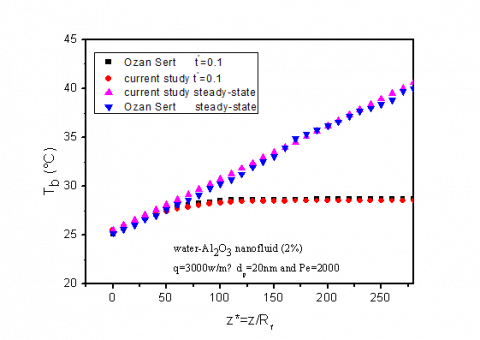Figure 2. Comparison of the obtained transient and study state bulk temperatures with those of Ozan Sert et al. 

Figure 2 shows a comparison of the axial distribution of the bulk nanofluid temperature for the above adopted boundaries and initial conditions, with negligible wall thickness. Excellent agreement between the results is observed at transient and steady state regimes. Similarly, in the same conditions, Figure 3 shows a comparison of the convective heat transfer coefficient of nanofluid along the tube, without wall thickness. There is a small relative difference between the current study and the values of Ozan Sert et al.  caused by their thermophysical properties which depend on temperature.

## 3.png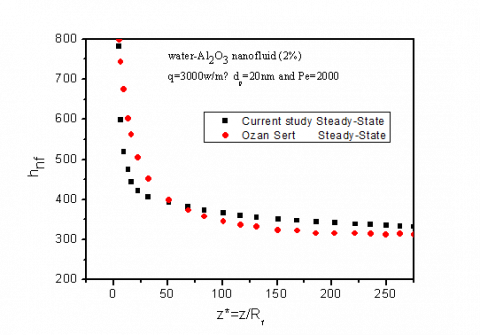Figure 3. Comparison between the convective heat transfer coefficient of Ozan Sert et al.  and the current study

## 4a.png(a)

## 4b.png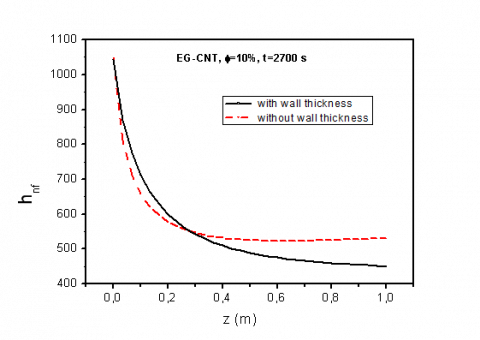(b)

## 4c.png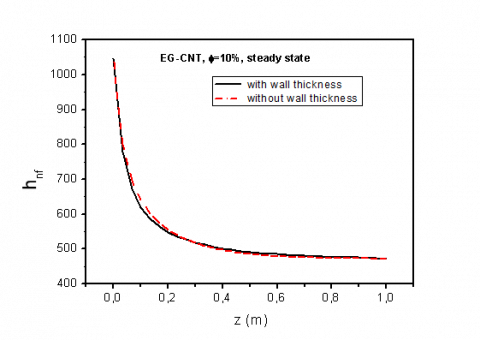(c)

Figure 4. Evolution of the convective heat transfer coefficient: (a) at 600 s, (b) at 2700 s, (c) Steady State

Figure 4 shows the convective heat transfer coefficient along the tube with or without wall thickness at different times (t=600s: Figure 4a, t=2700s: Figure 4b, Steady state: Figure 4c) at a given nanoparticle volume fraction ( $\phi=10 \%$ ).

As a result, the time has a significant effect on the convective heat transfer. At t=600s, fig 4a there is an important difference between the two cases: with and without wall thickness during the first instants of the transient regime, but at t=2700 s, fig 4b, it decreases as it approaches the steady state, fig 4c. This difference is principally due to the temperature gradient in the interface (nanofluid-steel wall) and the gap between the interfacial wall-fluid temperature and average bulk nanofluid one.

3. Results and Discussion

In this study, unsteady laminar forced convection heat transfer of nanofluids in cylinder is investigated. Numerical simulations have been performed by using the following data:

· Geometry of the duct:

$L=1 \quad m$ , $U_{\operatorname{moy}}=2.47 .10^{-2} \mathrm{m} s^{-1}$ , $R_{f}=5 m m$ , $R_{s}=2 m m$

· Physical properties of the duct:

$\lambda_{s}=40 \mathrm{W} / \mathrm{mC}$ , $\alpha_{s}=310^{-7} \mathrm{m} / \mathrm{s}$

· Initial and boundary conditions:

$T_{0}=293 \mathrm{K}$ , $T_{e}=293 K$ , $T_{a}=293 \mathrm{K}$ , $q_{0}=5000 \mathrm{W} / \mathrm{m}^{2}$ , $\omega=3.10^{-3} r d / s$ , Pe=1000.

· -Discretization:

$\Delta t=1 s$ , $N_{t}=7200$ , $N_{r f}=51$ , $N_{r p}=11$ , $N_{z}=31$ .

· Physical properties of the nanofluid and hybrid nanofluid: see tables 1 and 2.

Table 1. Thermophysical properties of base fluid and nanofluid

 EG 10% EG/CNT Kg.m 3 998,3 1038.5 W .m 1K 1 0.602 0.8026 C p J.kg 1K 1 4182.2 3901.98 E  Pa..s 1 1,001.10-3 1,25.10-3 Pr 6.954 4.868

Table 2. Thermophysical properties of nanoparticles and hybrid nanofluid

 CNT Ag EG/CNT-Ag Kg.m 3 1400 10500 1133.5 W .m 1K 1 3500 429 0.825 C p J .kg 1K 1 1380 235 3862.5 Pa..s 1 - - 1.25 10-3

3.1 Physical aspects

The volume fraction has an effect on many additional parameters like, thermal conductivity, dynamic viscosity and pression drop. In this work, the effect of the pression drop is low because we supposed the flow is established. Figure 5 shows the variation of the Prandtl number with the nanoparticle volume fraction for two studied nanofluids (EG/CNT and EG/Al2O3). The choice of EG as base fluid is referred to the fact that it leads to a higher exchange of heat transfer as it was mentioned in literature. It is useful to recall that the EG/CNT Prandtl number is higher than EG/Al2O3 one, mostly for $\phi>4 \%$ .

## 5.pngFigure 5. Variation of the Prandtl number with thenanoparticle volume fraction for two studied nanofluids (EG/CNT and EG/Al2O3)

## 6.png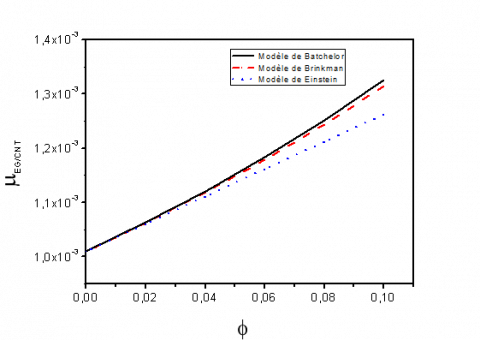Figure 6. Dynamic viscosity of nanofluid for three models versus nanoparticle volume fractions

## 7.png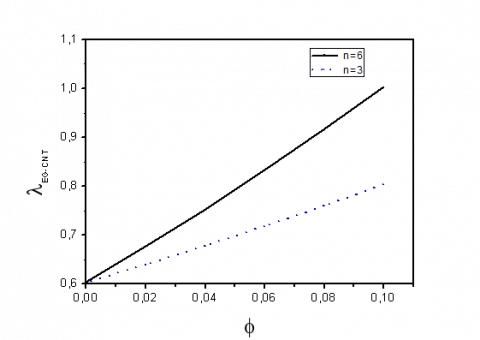Figure 7. Thermal conductivity of nanofluid versus nanoparticle volume fractions for cylindrical (n=6) and spherical nanoparticles (n=3)

For Einstein, Brinkman and Batchelor classical models, Figure 6 shows the evolution of EG/CNT nanofluid dynamic viscosity versus nanoparticle volume fraction. As it is depicted, between $\phi=0 \%$ and $\phi=4 \%$ , the increase of the dynamic viscosity is identical for all models. But for $4 \% \leq \phi \leq 10 \%$ , Einstein model which is retained in our study, gives lower values of $\mu$ compared to the two others models.

Figure 7 shows the effect of CNT nanoparticles shape on EG/CNT nanofluid thermal conductivity. It can be concluded that the shape of nanoparticles has an effect on the thermal conductivity. The cylindrical nanoparticles have higher conductivity than the spherical nanoparticles.

3.2 Thermal aspects

3.2.1 Uniform heat flux

3.2.1.1 Temperature profiles

The radial profile of the nanofluid temperature of EG/CNT at three axial positions （ $z=L / 3,2 L / 3, L$ ）is reported at different times in Figures.8a and 8b.

The thickness of the wall and its thermal conductivity make that there is no significant effect on the radial variation on the temperature of the wall.

Figure 9 shows the development of temperature profiles of nanofluid and steel wall of the tube. Given the fact that the nanofluid receives continuously a heat flux by the tube wall, its temperature continues to increase with z. The nanofluid bulk temperature is much lower at short time than at the steady state regime. By following the evolution of the tube wall temperature as a function of z, it is concluded that $\frac{\partial T_{s}}{\partial z} \neq 0$ whatever the time. As it is depicted in Figure 10, for a given time, the nanofluid bulk temperature increases with z. For a given location, it increases for $0<t<t_{e}$ where $t_{e}$ is the time at which the thermal regime becomes established. This time also increases with axial positions.

## 8.png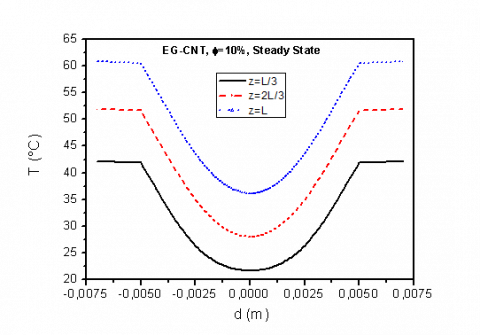Figure 8a. Steady state radial profiles of temperature at different axial positions

## 8b.png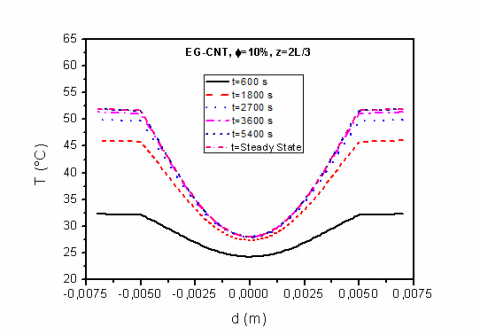Figure 8b. Radial profiles of temperature at different instants

## 9.pngFigure 9. Transient distributions of bulk temperature of nanofluid and wall temperature

## 10.png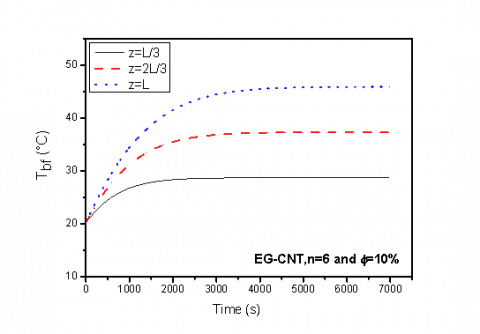Figure 10. Evolution of bulk nanofluid temperature for different axial positions

3.2.1.2 Convective heat transfer coefficient profiles

Figure 11 shows the axial profile of the convective heat transfer coefficient for different times. It can be seen that the heat transfer coefficient slightly increases with time and decreases along the tube length. Also, as expected, a significant increase of the transfer coefficient is observed for EG/CNT nanofluid in comparison with that obtained with EG pure. This increasing is explained by the augmentation of the thermal conductivity.

Figure 12 illustrates a comparison between the effects of cylindrical particles and the spherical particles on convective heat transfer coefficient. Mostly for z>0.18 m, it is clearly observed, that the cylindrical shape (n=6) leads to higher values of $h_{n f}$ than those of spherical shape (n=3). This type of development is intimately linked to the thermal conductivity evolution shown in Figure 7.

## 11.pngFigure 11. Transient axial profiles of the convective heat transfer coefficient for Ethylene Glycol and EG-CNT

## 12.png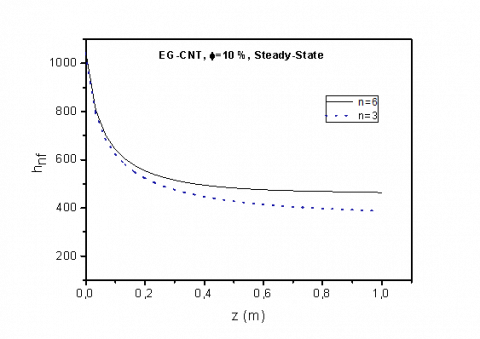Figure 12. Convective heat transfer coefficient forcylindrical (n=6) and spherical nanoparticles (n=3)

For EG/CNT nanofluid, we present in Figure 13 the axial distribution of the convective heat transfer coefficient, for different nanoparticle volume fractions and in Figure 14, its evolution for the mentioned axial positions. As it is seen, this coefficient increases with $\phi$ , and the steady state regime is reached at time $t=5400 s$. From Figure 10, it is possible to conclude that nanofluid with higher nanoparticle volume fractions has more ability to exchange heat transfer. The values of the convective heat transfer coefficient slightly decrease with an increase in the axial position.

For $\phi=10 \%$ , Figure 15 presents a comparison between the convective heat transfer coefficient for EG/CNT and EG/CNT-Ag. As is expected, higher heat transfer coefficient is observed for hybrid nanofluid. This is due to the higher value of thermal conductivity of hybrid nanofluid compared to sample nanofluid.

## 13.png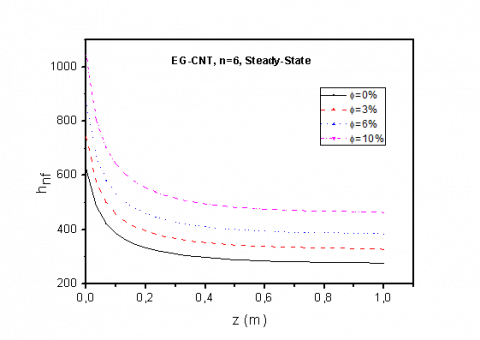Figure 13. Convective heat transfer coefficient for different nanoparticle volume fractions

## 14.pngFigure 14. Evolution of heat transfer coefficient of EG-CNTnanofluid for different axial positions

## 15.png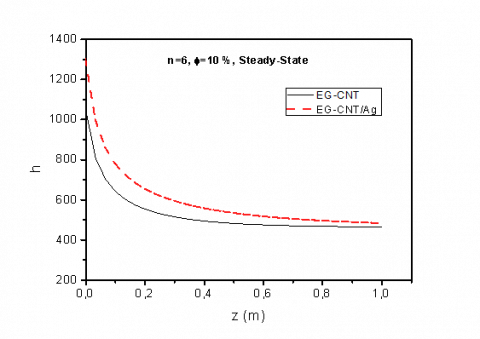Figure15. Distribution of convective heat transfer coefficient for EG-CNT and EG/CNT-Ag

3.2.2 Variable heat flux

The imposed wall density of heat flux is given by the following periodic form:

$q(t)=q_{0}(1+\sin (\omega t))$ , $\omega$ =3×10-3 rad /s , $q_{0}=5000 \quad W / m$

3.2.2.1 Temperature profiles

## 16.pngFigure 16. Variation of the temperature versus time at two axial positions

Figure16 shows the evolution of the nanofluid bulk temperature and steel wall temperature versus time at two axial positions. It can be seen that the nanofluid bulk temperature oscillates with time and increases with z for a given time. At initial times, the values of the temperature slightly increase with axial position. After a time, response of one hour, the nanofluid bulk temperature oscillates with the quasi-same magnitude. It should be noted that the nanofluid bulk temperature shows a similar behavior as the tube wall temperature versus the time.

For different thicknesses and for z=2L/3, figure 17 shows the evolution of the average temperature of EG base fluid and EG-CNT nanofluid. We observe the presence of the reduction of thermal amplitude with the thickness, this is due to the role of the thermal inertia which has for main role to oppose the variation of the temperature.

It is concluded that whatever the thickness value the presence of nanoparticles in the base fluid causes a thermal phase shift.

## 17.png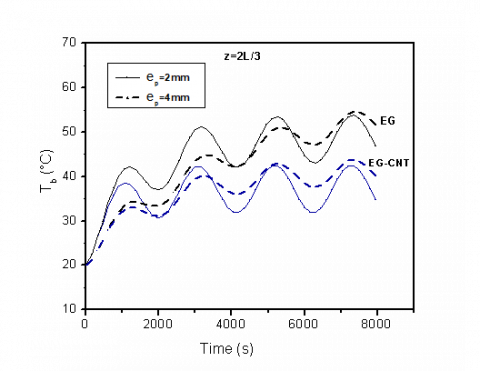Figure 17. Evolution of the average temperature of EG and EG-CNT nanofluid for two wall thicknesses

3.2.2.2 Convective heat transfer coefficient profiles

Figure 18 shows the axial convective heat coefficient for different volume fraction nanoparticles. The same conclusion, with an imposed constant flux, is observed. Figure 19 illustrates the evolution of the heat transfer coefficient of nanofluid with time at different axial positions. At the first instants, the values of the heat transfer coefficient are significantly higher and decrease rapidly. After that, they begin to oscillate with amplitudes which diminish gradually as one approaches the exit of the tube.

## 18.png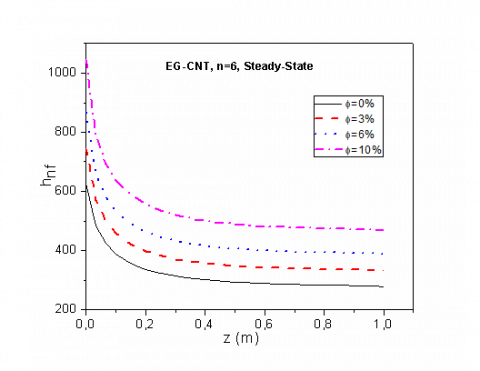Figure 18. Convective heat transfer coefficient for different nanoparticle volume fractions

## 19.png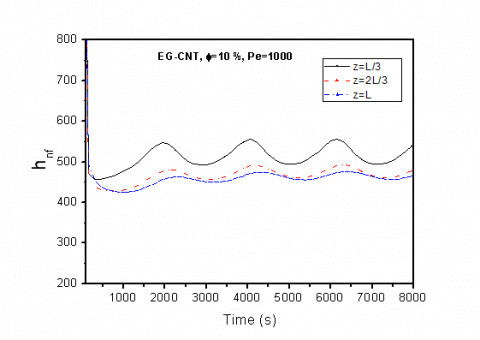Figure 19. Evolution of heat transfer coefficient of EG-CNT nanofluid for different axial positions

3.2.2.3 Thermal phase shift profiles

The thermal phase shift between two temperatures peaks is expressed by $\Delta \varphi=\omega \Delta t$ , where $\omega$ is the angular frequency of the imposed wall heat flux and $\Delta t$ is the time difference between two peaks. It principally depends of the layer thickness and the thermphysical properties of the material.

Figure 20 shows the time evolution of interfacial temperature at z=L for EG and EG-CNT nanofluid for two Peclet number, Pe=1000 and Pe=2000 at ep=2 mm, where the thermal phase shift is caused by the moving nanofluid and by steel layer of the tube.

## 20.png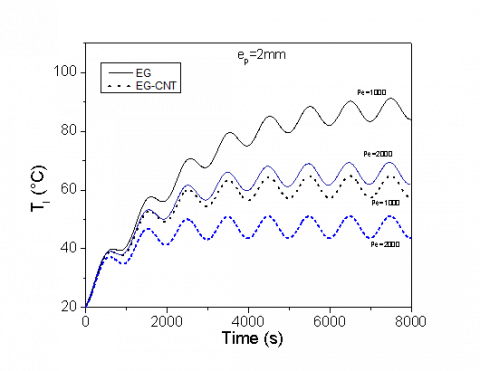Figure 20. Time evolution of interfacial temperature at z=L for EG and EG-CNT nanofluid

## 21.png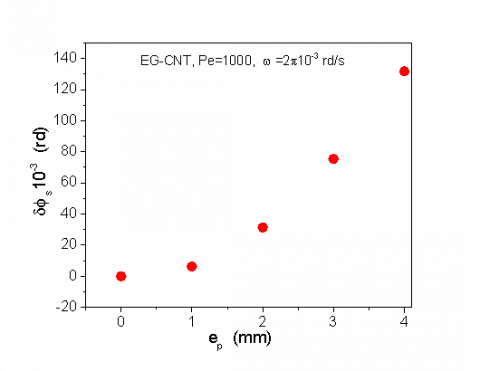Figure 21. Wall thermal phase shift of EG-CNT nanofluid at different thickness

Figure 21 shows the wall thermal phase shift at different thickness of nanofluid at a fixed time t=1000 s and Peclet number Pe=1000. As it is depicted the thermal phase shift increased with the increasing of the wall thickness.

4. Conclusion

In this work, a numerical study of laminar conjugated heat transfer mechanisms of EG/CNT nanofluid and EG/CNT-Ag hybrid nanofluid inside a heated horizontal tube is proposed to improve the efficiency of an absorber tube of the solar collector. The main conclusions, from the discussed results, are drawn as follow:

-The dynamic viscosity for the three models

(Einstein, Brinkman and Batchelor) and the thermal conductivity of Hamilton&Crosser model increases with the nanoparticles volume fractions.

-The shape of nanoparticles has an effect on the thermal conductivity which is higher for cylindrical particles than spherical particles.

-Whatever the wall heated flux nature (uniform or variable), convection heat transfer coefficient increases with nanoparticles volume fraction.

-Nanofluid bulk temperature increases with the axial direction and with time.

-For a given position, the heat transfer coefficient hnf increases versus time.

-A phase shift appears in the presence of nanoparticles in the base fluid.

$h_{E G / C N T-A g}>h_{E G / C N T}>h_{p u r e} E G$

This work has not been finished, so a continuous study on the radiation phenomenon of the solar collector seems to be necessary, to find a comparison between the efficiency of the solar collectors based water and based nanofluid.

Nomenclature
 CP specific heat, J. kg-1. K-1 d diamter of the tube, m dp diamter of the nanoparticle, m ep ep=Rp-Rf h heat transfer coefficient,W. m-2.K-1, U velocity,m.s-1 L lenght of the tube, m n shape factor N number of nodes Nu Nusselt number Pe Peclet number q(t) transient heat flux, q0 constant heat flux, W.m-2 r radial coordianate, m Rf internal radius, m Rp external radius, m T temperature, K Ta ambient temperature, K Te inlet temperature, K Ts wall temperature, K T0 initial temperature, K t time, s z axial coordinate EG Ethylene Glycol CNT Carbon nanotube Ag Argent Al2O3 Alumina Greek Symbols a thermal diffusivity, m2. s-1 f nanopartcile volume fraction µ dynamic viscosity, kg. m-1.s-1 $\lambda$ thermal conductivity, W.K-1.m-1 $\rho$ density, Kg.m-3 $\psi$ nanoparticle sphericity $\varphi$ thermal lag (phase shift) $\omega$ angular frequency Subscripts np nanoparticle f fluid s solid nf nanofluid e established thermal regime
References

 Kakac S., Pramuanjaroenkij A. (2009). Review of convective heat transfer enhancement withnanofluids, Internatioal Journal of Heat and Mass Transfer, Vol. 52, pp. 3187-3196. DOI: 10.1016/j.ijheatmasstransfer.2009.02.006

 Xan Y., Li Q. (2003), Investigation on convective heat transfer and flow features of nanofluids, Journal of Heat Transfer, pp. 125-151. DOI: 10.1115/1.1532008

 Slaman B.H., Mohammed H.A., Shkerbeet A. (2012). Heat transfer enhancement of nanofluids flow in microtube with constant heat flux, International Communications in Heat and Mass Transfer, p. 39. DOI: 10.1016/j.icheatmasstransfer.2012.07.005

 Choi S.U.S., Zhang Z.G., Yu W., loockwood F.E., Grulke E.A. (2001). Anomalous thermal conductivity enhancement suspension, Applied Physics Letters, pp. 79. DOI: 10.1063/1.1408272

 Xuan Y., Roetzel W. (2000). Conception of heat transfer correlation of nanofluids, International Journal of Heat and Mass Transfer, Vol. 43, pp. 3701-3707. DOI: 10.1016/S0017-9310(99)00369-5

 Maxwell J.C. (1873). Electricity and magnetism, Clarendon Press, Oxford, UK.

 Maxwell J.C. (1881). ATreatise on electricity and magnetism, Oxford University Press, Cambridge, UK, 2nd edition.

 Choi S.S. (1995). Enhancing thermal conductivity of fluids with nanoparticles, Energy Technology Division and Materials Science Division, Agronne National Laboratory.

 Pak B.C., Cho Y.I. (1998). Hydrodynamic and heat transfer study of dispersed fluids with submicron metallic oxide particles, Experimental Heat Transfer, Vol. 11, pp. 151-170. DOI: 10.1080/08916159808946559

 Heris S.Z., Etemad S.G., Esfahany M.N. (2006). Experimental investigation of oxide nanofluids laminar flow convective heat transfer, International Communications of Heat and Fluid Flow, Vol. 33. DOI: 10.1016/j.icheatmasstransfer.2006.01.005

 Heris S.Z., Esfahany M.N., Etemad S.G. (2007). Experimental investigation of convective heat transfer of Al2O3/water nanofluid in circular tube, International Journal of Heat and Fluid Flow, Vol. 28, pp. 203-210. DOI: 10.1016/j.ijheatfluidflow.2006.05.001

 Heris S.Z., Esfahany M.N., Etemad S.G. (2007). Numerical investigation of nanofluid laminar convective heat transfer through a circular tube, Numerical Heat Transfer, Part A, Vol. 52, pp. 1043-1058. DOI: 10.1080/10407780701364411

 Onticar P., Prasher R., Taylor R.A. (2010). Nanofluid based direct absorption solar collector, Journal of Renewable and Sustainable Energy, Vol. 2. DOI: 10.1063/1.3429737

 Yousefi T., Veysi F., Shojaeizadeh E., Zinadini S. (2012). An experimental investigation on the effect of Al2O3-H2O nanofluid on the efficiency of flat-plate solar collectors, Renewable Energy, Vol. 39, pp. 293-298. DOI: 10.1016/j.renene.2011.08.056

 Lenert A., Wang E.N. (2012). Optimization of nanofluid volumetric receivers for solar thermal energy conversion, Solar Energy, Vol. 86, pp. 253-65. DOI: 10.1016/j.solener.2011.09.029

 Maiga S.E.L.B., Nuyen C.T., Galanis N., Roy G., Maré T., Coqueux M. (2006). Heat transfer enhancement in turbulent tube using Al2O3 nanoparticle suspension, International Journal of Numerical Methods for heat & Fluid Flow, Vol. 16, pp. 275-292. DOI: 10.1108/09615530610649717

 Maiga S.E.l., Palm S.J., Nguyen C.T., Roy G., Galanis N. (2005). Heat transfer enhancement by using nanofluids in forced convection flows, International Journal of Heat and Mass Transfer, Vol. 26, pp. 530-546. DOI: 10.1016/j.ijheatfluidflow.2005.02.004

 Izadi M., Behzadmehr A., Jalali-Vahida, D. (2009). Numerical study of developing laminar forced convection of a nanofluid in an annulus, International Journal of Thermal Sciences, Vol. 48, pp. 2119-2129.

 Labib M.N., Nine M.J., Afrianto H., Chung H., Jeong H. (2013). Numerical investigation on effect of base fluids and hybrid nanofluid in forced convective heat transfer, International Journal of Thermal Sciences, Vol. 71, pp. 163-171. DOI: 10.1016/j.ijthermalsci.2009.04.003

 Moghadassi A., GHomi E., Parvizian F. (2015). A Numerical study of water based Al2O3 and Al2O3-Cu hybrid nanofluid effect on forced convective heat transfer, International Journal of Thermal Sciences, Vol. 92, pp. 50-57, DOI: 10.1016/j.ijthermalsci.2015.01.025

 Fakoor-Pakdman M., Amdisheh-Tadbir M., Bahrami M. (2014). Unsteady laminar forced convective tube flow under dynamic time dependent heat flux, Journal of Heat Transfer, Vol. 136. DOI: 10.1115/1.4026119

 Mahbubul I.M., Chong T.H., Khalduzzaman S.S., Shahrul I.M., saidur R., Long B.D., Amalina M.A. (2014). Effect on ultrasonication duration on colloidal structure and viscosity of alumina-water nanofluid, I&EC Research, Vol. 53, pp. 6677-6684. DOI: 10.1021/ie500705

 Duangthongsuk W., Wongwises S. (2010). Comparison of the effects of measured and computed thermophysical properties of nanofluids on heat transfer performance, Experimental Thermal and Fluid Sciences, Vol. 34, pp. 616-624. DOI: 10.1016/j.expthermflusci.2009.11.012

 Takabi B., Salehi S. (2014). Augmentation of the heat transfer performance of a sinusoidal corrugated enclosure by employing hybrid nanofluid, Hindawi Publishing Corporation Advances in Mechanical Engineering. DOI: 10.1155/2014/147059

 Ozan S.I., Sezer U.N., Kakaç S. (2013). Numerical analysis of unsteady laminar forced convection of nanofluids in circular ducts, Heat Mass Transfer, Vol. 49, pp. 1405-1417. DOI: 10.1007/s00231-013-1184-1